menu 3 Newton's Law of GravitationErasmus+: Key action 2:Strategic Partnerships in School Education# INTRODUCTION

Johannes Kepler postulated his laws for the motion of the Solar system planets based on observational data. But his model did not explain WHY the planets move the way they do. The explanation was given by Sir Isaac Newton in the second half of the 17 century in his law of universal gravitation. This law introduces a new force – the gravitation, which not only explains the motions of the celestial objects, but also makes the objects fall to the Earth’s surface.

## Contents

In the part for the teacher in this section, we will first describe Newton’s law of gravitation, then see how this law, together with other of Newton’s laws for motion generalize Kepler’s laws, how particular velocities in space are defined and what is their meaning. In the end, we will look at the concepts of mass and weight and what is the difference between them. In the part with practical exercises for the students we include several interesting problems and questions which aim to illustrate the theoretical material and help for its better understanding.

# Newton's Law of Gravitation

Let us consider the Sun – Earth system. According to Newton’s law of gravitation, the Earth feels a gravitational pull, which is proportional to the product of the masses of the Earth and the Sun and is inversely proportional to the square of the distance between their centres.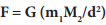where G is the gravitational constant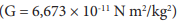, m1 – is the mass of the Earth,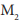– is the mass of the Sun, d – is the distance between their centres.

An interactive representation of the gravitational force between a star and a planet can be found here: https://www.physicsclassroom.com/Physics-Interactives/Circular-and-Satellite-Motion/Gravitational-Fields/Gravitational-Fields-Interactive.

At first Newton postulates the law as: “The force that makes the planets move along elliptical orbits around the Sun is always directed towards the Sun (i.e it is a central force) and is inversely proportional to the square of the distance between the planet and the sun.” Later he generalises: “Every point mass attracts every single other point mass by a force acting along the line intersecting both points and the force is proportional to the product of the two masses and inversely proportional to the square of the distance between them”.

Since the gravitational force is always directed along the line intersecting both points, we can apply the law for a body falling to the ground. Then, in the equation for the gravitational force, m 1 will be the mass of the falling object, М2 will be the Earth’s mass, and d will be the radius of the Earth. But according to Newton,s law of motion the force acting on the object will be equal to the product of its mass and the acceleration it receives. Then: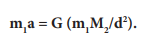So we can express the acceleration of a falling object as: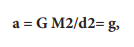g is called gravitational acceleration (or Earth's gravity, or free-fall acceleration). It is approximately 9,8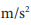on average. It is imparted to all objects in proximity to the Earth. Sometimes 9,8is also called standard gravity.

Newton’s laws for gravity and motion can be used to generalise Kepler’s law for planetary motion:

1. The orbits of two bodies, moving around a common centre of mass under the force of gravitation are always described by one of the conic sections – an ellipse, a parabola, or a hyperbola.

2. The line, connecting the two bodies will sweep out equal areas during equal intervals of time. This law suggests that the motion of the bodies along their orbit will be faster when they are closer to the centre of mass, and slower when they are farther away from it.

3. This law is about the relative orbit of one body around the other. Then, the sum of the masses of the two bodies, multiplied by the square of the orbital period of the moving body is equal to the cube of the semi-major axis of its orbit:: (m1 + m2 ) P2 = a3. This expression is particularly useful for obtaining the masses of stars.

filter_none